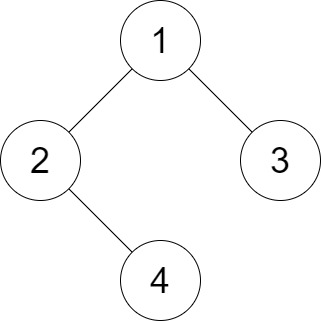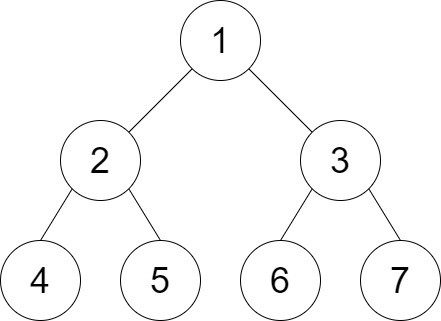# GeetCode Hub

Given the `root` of a binary tree and an integer `distance`. A pair of two different leaf nodes of a binary tree is said to be good if the length of the shortest path between them is less than or equal to `distance`.

Return the number of good leaf node pairs in the tree.

Example 1:```Input: root = [1,2,3,null,4], distance = 3
Output: 1
Explanation: The leaf nodes of the tree are 3 and 4 and the length of the shortest path between them is 3. This is the only good pair.
```

Example 2:```Input: root = [1,2,3,4,5,6,7], distance = 3
Output: 2
Explanation: The good pairs are [4,5] and [6,7] with shortest path = 2. The pair [4,6] is not good because the length of ther shortest path between them is 4.
```

Example 3:

```Input: root = [7,1,4,6,null,5,3,null,null,null,null,null,2], distance = 3
Output: 1
Explanation: The only good pair is [2,5].
```

Example 4:

```Input: root = , distance = 1
Output: 0
```

Example 5:

```Input: root = [1,1,1], distance = 2
Output: 1
```

Constraints:

• The number of nodes in the `tree` is in the range `[1, 2^10].`
• Each node's value is between `[1, 100]`.
• `1 <= distance <= 10`

/** * Definition for a binary tree node. * public class TreeNode { * int val; * TreeNode left; * TreeNode right; * TreeNode() {} * TreeNode(int val) { this.val = val; } * TreeNode(int val, TreeNode left, TreeNode right) { * this.val = val; * this.left = left; * this.right = right; * } * } */ class Solution { public int countPairs(TreeNode root, int distance) { } }